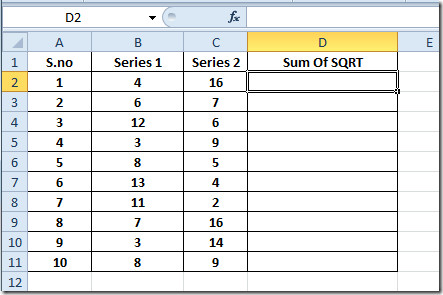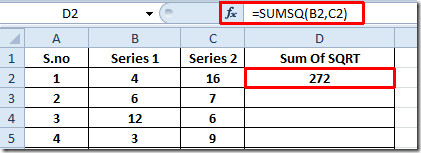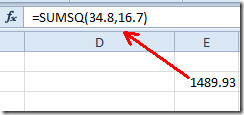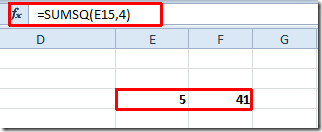# Excel 2010: SUMSQ function

Excel SUMSQ is very useful function when you need to return the sum of squares of the specified values in spreadsheet, rather than going for manual evaluation. It would be more useful by providing it with location of cells as function arguments where the data is residing, but you can directly put in values as argument to get them evaluated.

Launch Excel 2010 spreadsheet on which you want to apply SUMSQ function. For instance, we have included a spreadsheet containing fields, S.No, Series 1, Series 2 and Sum of SQRT.Now we will find out the square of both values in Series 1 and Series 2 field and show sum of the resultant values. For this we will be using SUMSQ function.

The syntax of SUMSQ function is;

=SUMSQ(number1, number2…)

We will be putting location of cells as arguments in the function and write this function in first row of SUM Of SQRT field as;

=SUMSQ(B2,C2)

The function arguments B2 and C2 are the location of cells. It will yield SUM of values after taking square of both values, as shown in the screenshot below.Drag the plus sign at the end of the cell towards the end of column to apply it over the Sum Of SQRT field.If you want, you can evaluate SUMSQ function exclusively by putting in values directly.Now for using this function by giving direct value and location of cells simultaneously, you will need to write it as;

=SUMSQ(location_of_cell, number)You can also check out previously reviewed Excel function; DOLLAR, SUMPRODUCT, SUMIF, COUNTIF, VLOOKUP, HLOOKUP ,PMT, and LEN.

This site uses Akismet to reduce spam. Learn how your comment data is processed.